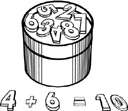Also found in: Thesaurus, Medical, Legal, Encyclopedia, Wikipedia.

(ăd′ĕnd′, ə-dĕnd′)
n.
Any of a set of numbers to be added.

(ˈædɛnd; əˈdɛnd)
n
(Mathematics) any of a set of numbers that is to be added. Compare sum11

(ˈæd ɛnd, əˈdɛnd)

n.
any of a group of numbers or terms added together to form a sum.

(ăd′ĕnd′)
A number that is added to another number.
ThesaurusAntonymsRelated WordsSynonymsLegend:
 Noun 1addend - a number that is added to another number (the augend)number - a concept of quantity involving zero and units; "every number has a unique position in the sequence"
Translations
sčítanec

n (US) → Summand m
Mentioned in ?
References in periodicals archive ?
Observe the way one (randomly chosen) addend (the variable called 'firstnumber') is combined with another to create a total sum--which is to be the correct answer for an addition question.
Blau's Index calculated the amount of ingroup diversity by adding the squared percentage of individuals in an identified category, summing the proportions, and then subtracting the addend from one.
The addend of 0.8*P was increased to 1.1*P because of the doubled run-in of the thread by using the additional design element wired thread insert.
The second addend at the RHS of (46) identically vanishes as it can be reduced to be a surface integral of P over [partial derivative]{[xi]}, where P [equivalent to] 0.
The theme of this excellent collection of essays becomes immediately apparent with the cover art, where the two words "text" and "field" stand around the plus in an uneasy relationship: While "text" has a certain pride of place in coming first, it is "field" in all caps that demands attention and dominates the frame, nearly threatening to overwhelm its purported addend. Text + Field: Innovations in Rhetorical Method explores a variety of methods for rhetorical critics interested in exploring the relationship between rhetoric and the sites in which it is produced, consumed, and, ultimately evaluated.
where [[PHI].sub.1] is an ad hoc addend in (2) and (3), which was added by Schroeder  empirically to obtain more appropriate values for the initial phases [[PHI].sub.i] of signal components enabling so to achieve the lowest CF.
This method is used in multi-bit parallel adders where the carry into an individual element of the adder shall be predicted by a smaller delay that is required to produce the carry by rippling through previous adder stages as the result of adding the less significant addend and augends bits.
Considering the envelope, the second addend in (23) can be ignored; that is, [tau] [approximately equal to] 2[R.sub.0]/C, and the expression (22) is written as
In the implementation, when using fma instruction, it is required that multiplicand a and multiplier b are both in Simplified format and addend c is in Redundant format but less than ([2.sup.53] - [2.sup.46]).
For example, using a school-based instructional program, Cihak and Grim (2008) initially taught participating students (n = 4) a skill-purchasing strategy involving "counting on," or adding sums beginning from the larger or smaller addend (e.g., 3 + 2 = 3, 4, 5) rather than counting all addends (e.g., 3 + 2 = 1, 2, 3, 4, 5).

Site: Follow: Share:
Open / Close